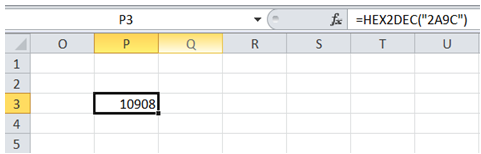## Convert hex values into decimal in Excel

For example, the value returned by SW is Hex "2A9C". In Excel, how do I convert this into Dec.

To do it in Excel, here is the answer:

a) Enter the formula =HEX2DEC("2A9C") in an empty cell as shown below. The formula returns Decimal value for the Hex input.

Note: The Hex value has to be entered within quotes.